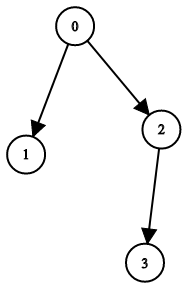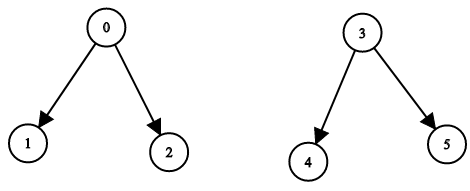Formatted question description: https://leetcode.ca/all/1361.html

# 1361. Validate Binary Tree Nodes (Medium)

You have n binary tree nodes numbered from 0 to n - 1 where node i has two children leftChild[i] and rightChild[i], return true if and only if all the given nodes form exactly one valid binary tree.

If node i has no left child then leftChild[i] will equal -1, similarly for the right child.

Note that the nodes have no values and that we only use the node numbers in this problem.

Example 1:Input: n = 4, leftChild = [1,-1,3,-1], rightChild = [2,-1,-1,-1]
Output: true


Example 2:Input: n = 4, leftChild = [1,-1,3,-1], rightChild = [2,3,-1,-1]
Output: false


Example 3:Input: n = 2, leftChild = [1,0], rightChild = [-1,-1]
Output: false


Example 4:Input: n = 6, leftChild = [1,-1,-1,4,-1,-1], rightChild = [2,-1,-1,5,-1,-1]
Output: false


Constraints:

• 1 <= n <= 10^4
• leftChild.length == rightChild.length == n
• -1 <= leftChild[i], rightChild[i] <= n - 1

Related Topics:
Graph

## Solution 1.

Firstly, we can check the in-dgree.

• Only one node (the root) has 0 in-degree. All other nodes have 1 in-dgree.

But this is not enough. For example:

[0,-1]
[-1,-1]


This graphy has one circle and one dangling node and satisfies the above requirement.

To rule this scenario out, we can add this requirement.

• If there are more than one node, the node with 0 in-degree must have out-degree.
// OJ: https://leetcode.com/problems/validate-binary-tree-nodes/
// Time: O(N)
// Space: O(N)
class Solution {
public:
bool validateBinaryTreeNodes(int n, vector<int>& leftChild, vector<int>& rightChild) {
vector<int> indegree(n);
for (int i = 0; i < n; ++i) {
int L = leftChild[i], R = rightChild[i];
if (L != -1 && indegree[L]++) return false; // if the indegree is more than 1, return false
if (R != -1 && indegree[R]++) return false;
}
int root = -1;
for (int i = 0; i < n; ++i) {
if (indegree[i]) continue;
if (root != -1) return false; // if more than one node with 0 indegree, return false
root = i;
}
return root != -1 && (n == 1 || leftChild[root] != -1 || rightChild[root] != -1); // root must exist, and the root must have out-degree unless there is only one node
}
};


Java

• class Solution {
public boolean validateBinaryTreeNodes(int n, int[] leftChild, int[] rightChild) {
int[] parents = new int[n];
Arrays.fill(parents, -1);
int edgesCount = 0;
for (int i = 0; i < n; i++) {
int curLeft = leftChild[i], curRight = rightChild[i];
if (curLeft >= 0) {
edgesCount++;
if (parents[curLeft] < 0)
parents[curLeft] = i;
else
return false;
}
if (curRight >= 0) {
edgesCount++;
if (parents[curRight] < 0)
parents[curRight] = i;
else
return false;
}
}
if (edgesCount != n - 1)
return false;
int root = -1;
for (int i = 0; i < n; i++) {
if (parents[i] == -1) {
if (root < 0)
root = i;
else
return false;
}
}
if (root < 0)
return false;
int visitCount = 0;
queue.offer(root);
while (!queue.isEmpty()) {
int node = queue.poll();
visitCount++;
int left = leftChild[node], right = rightChild[node];
if (left >= 0)
queue.offer(left);
if (right >= 0)
queue.offer(right);
}
return visitCount == n;
}
}

• // OJ: https://leetcode.com/problems/validate-binary-tree-nodes/
// Time: O(N)
// Space: O(N)
class Solution {
public:
bool validateBinaryTreeNodes(int n, vector<int>& leftChild, vector<int>& rightChild) {
vector<int> indegree(n);
for (int i = 0; i < n; ++i) {
int L = leftChild[i], R = rightChild[i];
if (L != -1 && indegree[L]++) return false; // if the indegree is more than 1, return false
if (R != -1 && indegree[R]++) return false;
}
int root = -1;
for (int i = 0; i < n; ++i) {
if (indegree[i]) continue;
if (root != -1) return false; // if more than one node with 0 indegree, return false
root = i;
}
return root != -1 && (n == 1 || leftChild[root] != -1 || rightChild[root] != -1); // root must exist, and the root must have out-degree unless there is only one node
}
};

• print("Todo!")